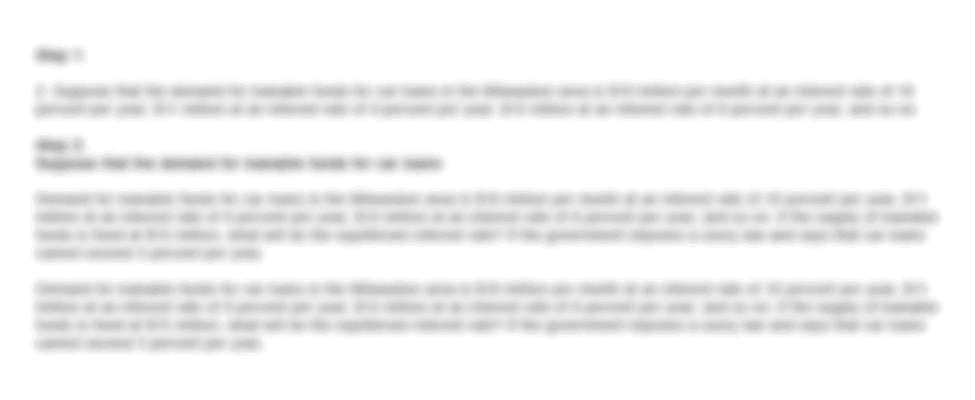• FAQ
• Contact/ Homework Answers / Finance / Suppose the interest rate one-year bonds equal to 7% Suppose
Not my Question
Flag Content

# Question : Suppose the interest rate one-year bonds equal to 7% Suppose

Suppose the interest rate on one-year bonds is equal to 7% Suppose one year from now the interest rate on one-year bonds is equal to 7.5% If the expectations theory holds, what is the interest rate on 2 year bonds? Why would the interest rate between two years vary? Explain

## Solution 5 (1 Ratings )

Solved
Finance 5 Months Ago 65 Views